# CSC321 Tutorial 6: Optimization and Convolutional Neural Networks¶

In lecture 5, we talk about different issues that may arise when training an artificial neural network. Today, we'll explore some of these issues, and explore different ways that we can optimize a neural network's cost function.

In lecture 6, we will cover convolutional neural networks. Since this is the last tutorial before reading week, we will also train some CNN's today. If you are in the Tuesday lecture section, don't worry! Think of CNN's as a neural network with a slightly different architecture, or that the weights are "wired" differently. These weights (parameters) still can be optimized via gradient descent, and we will still use the back-propagation algorithm.

Please note that because there is stochasticity in the way we initalize the neural network weights, so we will get different results (final training/validation accuracies) if we run the initialization + training multiple times. You will need to run some of the provided code multiple times to make a conclusion about what optimization methods work well.

In :
import matplotlib.pyplot as plt
import math
import torch
import torch.nn as nn
import torch.optim as optim

%matplotlib inline


## Data¶

We'll use the MNIST data set, the same data set that we introduced in Tutorial 4. The MNIST dataset contains black and white, hand-written (numerical) digits that are 28x28 pixels large. As in tutorial 4, we'll only use the first 2500 images in the MNIST dataset. The first time you run this code, we will download the MNIST dataset.

In :
from torchvision import datasets, transforms

mnist_train = datasets.MNIST('data',
train=True,
transform=transforms.ToTensor())
mnist_train = list(mnist_train)[:2500]

mnist_train, mnist_val = mnist_train[:2000], mnist_train[2000:]


## Models in PyTorch¶

We'll work with two models: a MLP and a convolutional neural network.

In :
# Multi-layer perceptron
class MLP(nn.Module):
def __init__(self, num_hidden):
super(MLP, self).__init__()
self.layer1 = nn.Linear(28 * 28, num_hidden)
self.layer2 = nn.Linear(num_hidden, 10)
self.num_hidden = num_hidden
def forward(self, img):
flattened = img.view(-1, 28 * 28) # flatten the image
activation1 = self.layer1(flattened)
activation1 = torch.relu(activation1)
activation2 = self.layer2(activation1)
return activation2

# You should understand this after Lecture 6
class CNN(nn.Module):
def __init__(self):
super(CNN, self).__init__()
self.conv1 = nn.Conv2d(in_channels=1,
out_channels=4,
kernel_size=3,
self.pool = nn.MaxPool2d(2, 2)
self.conv2 = nn.Conv2d(in_channels=4,
out_channels=8,
kernel_size=3,
self.fc = nn.Linear(8 * 7 * 7, 10)
def forward(self, x):
x = self.pool(torch.relu(self.conv1(x)))
x = self.pool(torch.relu(self.conv2(x)))
x = x.view(-1, 8 * 7 * 7)
return self.fc(x)


One way to gauge the "complexity" or the "capacity" of the neural network is by looking at the number of parameters that it has.

In :
def print_num_parameters(model, name="model"):
print("Number of parameters in %s" % name,
sum(p.numel() for p in model.parameters()))

print_num_parameters(MLP(200), "MLP(200)")
print_num_parameters(CNN(), "the CNN")

Number of parameters in MLP(200) 159010
Number of parameters in the CNN 4266


## Training the neural network¶

We'll use a fairly configurable training training function that computes both training and validation accuracy in each iteration. This is more

In :
def train(model, data, batch_size=64, weight_decay=0.0,
optimizer="sgd", learning_rate=0.1, momentum=0.9,
data_shuffle=True, num_epochs=10):
# training data
batch_size=batch_size,
shuffle=data_shuffle)
# loss function
criterion = nn.CrossEntropyLoss()
# optimizer
assert optimizer in ("sgd", "adam")
if optimizer == "sgd":
optimizer = optim.SGD(model.parameters(),
lr=learning_rate,
momentum=momentum,
weight_decay=weight_decay)
else:
lr=learning_rate,
weight_decay=weight_decay)
# track learning curve
iters, losses, train_acc, val_acc = [], [], [], []
# training
n = 0 # the number of iterations (for plotting)
for epoch in range(num_epochs):
for imgs, labels in iter(train_loader):
if imgs.size() < batch_size:
continue

model.train() # annotate model for training
out = model(imgs)
loss = criterion(out, labels)
loss.backward()
optimizer.step()

# save the current training information
iters.append(n)
losses.append(float(loss)/batch_size)             # compute *average* loss
train_acc.append(get_accuracy(model, train=True)) # compute training accuracy
val_acc.append(get_accuracy(model, train=False))  # compute validation accuracy
n += 1

# plotting
plt.title("Learning Curve")
plt.plot(iters, losses, label="Train")
plt.xlabel("Iterations")
plt.ylabel("Loss")
plt.show()

plt.title("Learning Curve")
plt.plot(iters, train_acc, label="Train")
plt.plot(iters, val_acc, label="Validation")
plt.xlabel("Iterations")
plt.ylabel("Training Accuracy")
plt.legend(loc='best')
plt.show()

print("Final Training Accuracy: {}".format(train_acc[-1]))
print("Final Validation Accuracy: {}".format(val_acc[-1]))


And of course, we need the get_accuracy helper function. To turn the probabilities into a discrete prediction, we will take the digit with the highest probability. Because of the way softmax is computed, the digit with the highest probability is the same as the digit with the (pre-activation) output value.

In :
def get_accuracy(model, train=False):
if train:
data = torch.utils.data.DataLoader(mnist_train, batch_size=4096)
else:
data = torch.utils.data.DataLoader(mnist_val, batch_size=1024)

model.eval() # annotate model for evaluation
correct = 0
total = 0
for imgs, labels in data:
output = model(imgs) # We don't need to run torch.softmax
pred = output.max(1, keepdim=True) # get the index of the max log-probability
correct += pred.eq(labels.view_as(pred)).sum().item()
total += imgs.shape
return correct / total


Let's see what the training curve of a multi-layer perceptron looks like. This code will take a couple minutes to run...

In :
model = MLP(50)
train(model, mnist_train, learning_rate=0.1)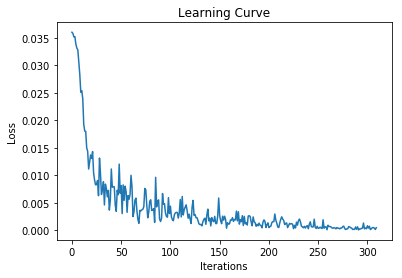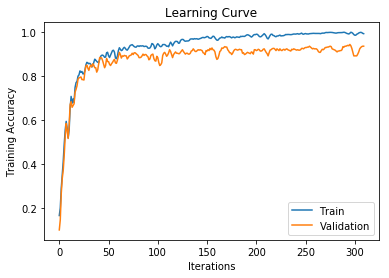Final Training Accuracy: 0.993
Final Validation Accuracy: 0.936


## MLP Hidden Unit Size¶

The first thing we'll explore is the hidden unit size. If we increase the number of hidden units in a MLP, we'll increase its parameters counts.

In :
print_num_parameters(MLP(50), "MLP with 50 hidden units")
print_num_parameters(MLP(100), "MLP with 100 hidden units")
print_num_parameters(MLP(200), "MLP with 200 hidden units")

Number of parameters in MLP with 50 hidden units 39760
Number of parameters in MLP with 100 hidden units 79510
Number of parameters in MLP with 200 hidden units 159010


With more hidden units, our model has more "capacity", and can learn more intricate patterns in the training data. Our training accuracy will therefore be higher. However, the computation time for training and using these networks will also increase.

Adding more parameters tend to widen the gap between training and validation accuracy. As we add too many parameters, we could overfit. However, we won't show that here since the computations will take a long time.

In :
model = MLP(100)
train(model, mnist_train, learning_rate=0.1)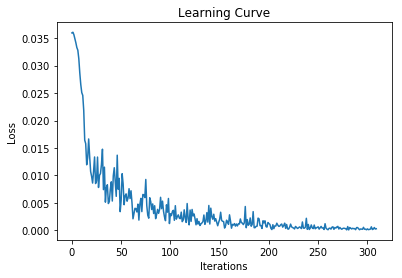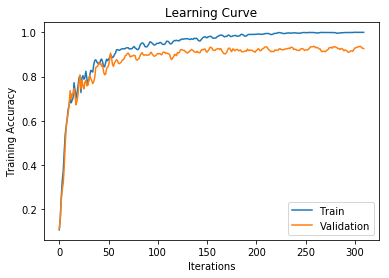Final Training Accuracy: 0.9995
Final Validation Accuracy: 0.926


A smaller network will train faster, but may have worse training accuracy. Bear in mind that since the neural networks initialization is random, k/

In :
model = MLP(30)
train(model, mnist_train, learning_rate=0.1)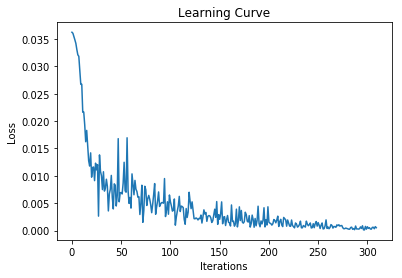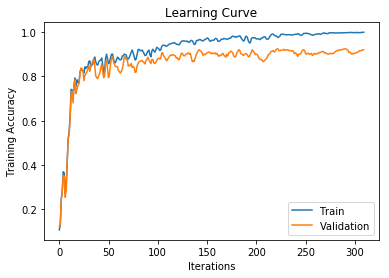Final Training Accuracy: 0.999
Final Validation Accuracy: 0.92


### Interlude: shuffling the dataset¶

What if don't off data_shuffle? That is, what if we use the same mini-batches across all of our epochs? Can you explain what's going on in this learning curve?

In :
model = MLP(30)
train(model, mnist_train, learning_rate=0.1, data_shuffle=False)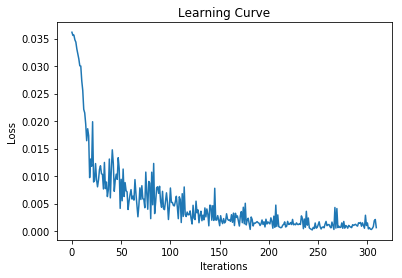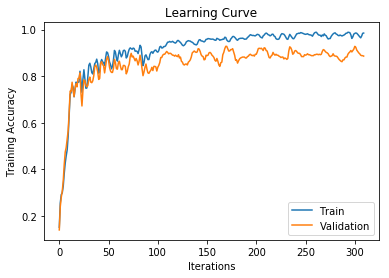Final Training Accuracy: 0.985
Final Validation Accuracy: 0.886


### Conv Net¶

The learning curve for the convolutional network looks similar. This network is a lot more compact with much fewer parameters. The computation time is a bit longer than training MLPs, but we get fairly good results. (The learning rate of 0.1 looks a little high for this CNN, based on the noisiness of the learning curves.)

In :
model = CNN()
print_num_parameters(model, "the CNN")
train(model, mnist_train, batch_size=64, optimizer="sgd", learning_rate=0.1,
momentum=0., num_epochs=5)

Number of parameters in the CNN 4266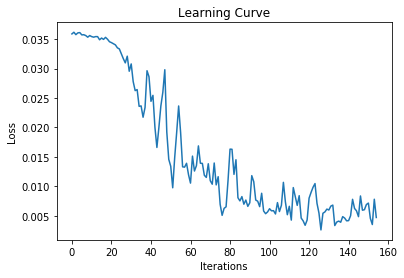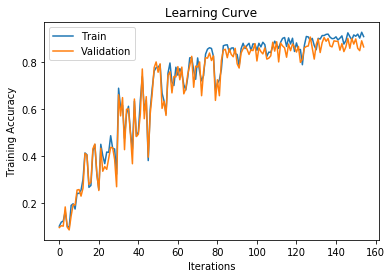Final Training Accuracy: 0.91
Final Validation Accuracy: 0.866


## Momentum¶

We'll mainly experiment with the MLP(30) model, since it trains the fastest. We'll measure how quickly the model trains by looking at how far we get in first 3 epochs of training. Here's how far our model gets without using momenutm, with a learning rate of 0.1.

In :
model = MLP(30)
train(model, mnist_train, learning_rate=0.1, momentum=0., num_epochs=3)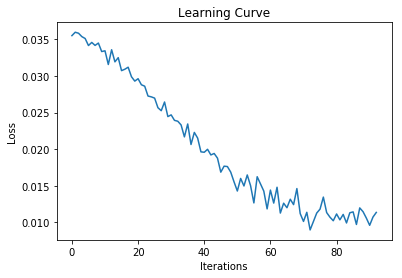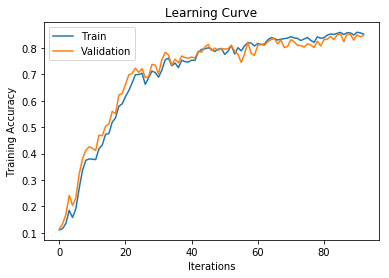Final Training Accuracy: 0.853
Final Validation Accuracy: 0.848


With a well-tuned learning-rate and momentum parameter, our training can go faster. (Note: We had to try a few settings before finding one that worked well, and encourage you to try different combinations of the learning rate and momentum. For example, learning rate of 0.1 and momentum of

In :
model = MLP(30)
train(model, mnist_train, learning_rate=0.05, momentum=0.9, num_epochs=3)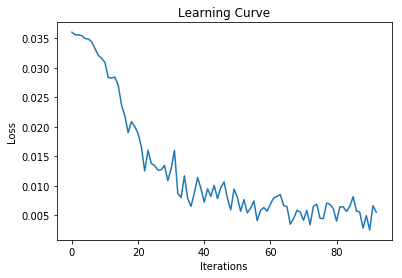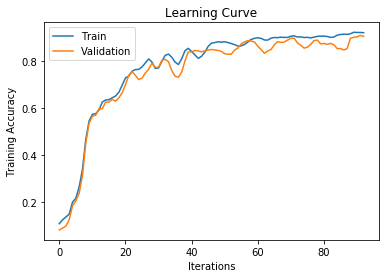Final Training Accuracy: 0.9195
Final Validation Accuracy: 0.904


The optimizer Adam works well and is the most popular optimizer nowadays. Adam typically requires a smaller learning rate: start at 0.001, then increase/decrease as you see fit. For this example, 0.005 works well.

In :
model = MLP(30)
train(model, mnist_train, optimizer="adam", learning_rate=0.005, num_epochs=3)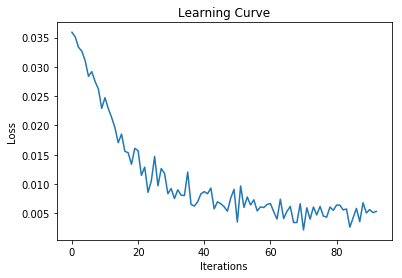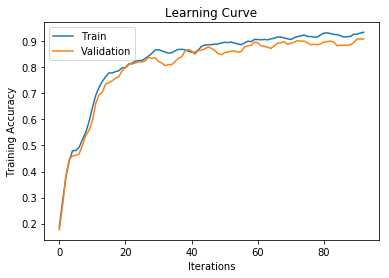Final Training Accuracy: 0.934
Final Validation Accuracy: 0.908


Convnets can also be trained using SGD with momentum or with Adam. In particular, our CNN generalizes very well. (Since our validation accuracy is about equal to our training accuracy, we can afford to increase the model capacity if we want to.)

In :
model = CNN()
train(model, mnist_train, optimizer="adam", learning_rate=0.005, num_epochs=3)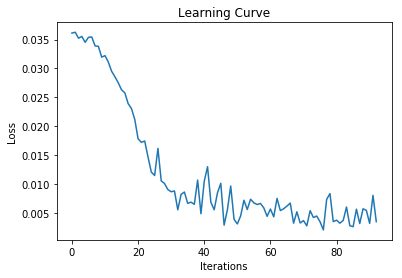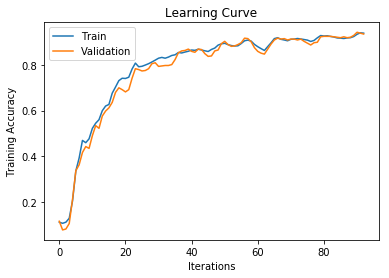Final Training Accuracy: 0.94
Final Validation Accuracy: 0.936

In :
# Uncomment to run
# train(CNN(), mnist_train, learning_rate=0.1, momentum=0.9, num_epochs=3)


## Batch Normalization¶

Batch normalization speeds up training significantly!

In :
class MLPBN(nn.Module):
def __init__(self, num_hidden):
super(MLPBN, self).__init__()
self.layer1 = nn.Linear(28 * 28, num_hidden)
self.bn = nn.BatchNorm1d(num_hidden)
self.layer2 = nn.Linear(num_hidden, 10)
self.num_hidden = num_hidden
def forward(self, img):
flattened = img.view(-1, 28 * 28) # flatten the image
activation1 = self.layer1(flattened)
activation1 = torch.relu(activation1)
activation1 = self.bn(activation1)
activation2 = self.layer2(activation1)
return activation2

mlp_bn = MLPBN(30)
train(mlp_bn, mnist_train, optimizer="adam", learning_rate=0.005, num_epochs=3)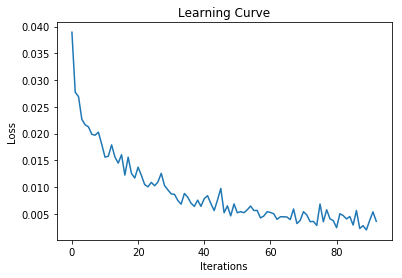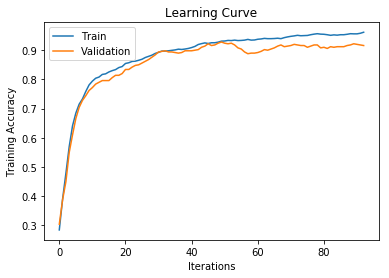Final Training Accuracy: 0.9615
Final Validation Accuracy: 0.916


There is a debate as to whether the batch-normalization should be applied before or after the activation. The original batch normalization paper applied the normalization before the ReLU activation, but applying normalization after the ReLU performs better in practice.

I (Lisa) believe the reason to be as follows:

1. If we apply normalization before ReLU, then we are effectively ignoring the bias parameter of those units, since those unit's activations gets centered anyways.
2. If we apply normalization after ReLU, we will have both positive and negative information being passed to the next layer.
In :
class MLPBNBeforeReLu(nn.Module):
def __init__(self, num_hidden):
super(MLPBNBeforeReLu, self).__init__()
self.layer1 = nn.Linear(28 * 28, num_hidden)
self.bn = nn.BatchNorm1d(num_hidden)
self.layer2 = nn.Linear(num_hidden, 10)
self.num_hidden = num_hidden
def forward(self, img):
flattened = img.view(-1, 28 * 28) # flatten the image
activation1 = self.layer1(flattened)
activation1 = self.bn(activation1)
activation1 = torch.relu(activation1)
activation2 = self.layer2(activation1)
return activation2

train(MLPBNBeforeReLu(30), mnist_train, optimizer="adam", learning_rate=0.005, num_epochs=3)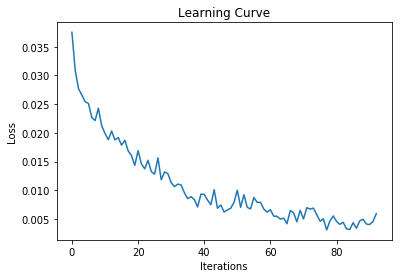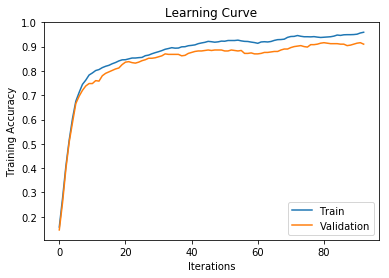Final Training Accuracy: 0.959
Final Validation Accuracy: 0.91


Batch normalization can be used in CNNs too.

In :
class CNNBN(nn.Module):
def __init__(self):
super(CNNBN, self).__init__()
self.conv1 = nn.Conv2d(in_channels=1,
out_channels=4,
kernel_size=3,
self.bn1 = nn.BatchNorm2d(4) # num out channels
self.pool = nn.MaxPool2d(2, 2)
self.conv2 = nn.Conv2d(in_channels=4,
out_channels=8,
kernel_size=3,
self.bn2 = nn.BatchNorm2d(8) # num out channels
self.fc = nn.Linear(8 * 7 * 7, 10)
def forward(self, x):
x = self.bn1(torch.relu(self.conv1(x)))
x = self.pool(x)
x = self.bn2(torch.relu(self.conv2(x)))
x = self.pool(x)
x = x.view(-1, 8 * 7 * 7)
return self.fc(x)

train(CNNBN(), mnist_train, optimizer="adam", learning_rate=0.005, num_epochs=3)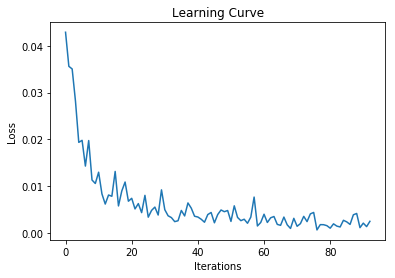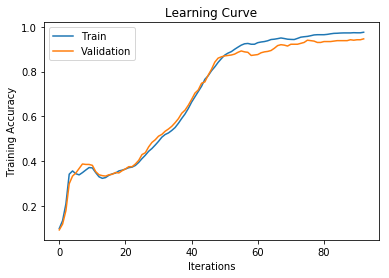Final Training Accuracy: 0.9755
Final Validation Accuracy: 0.946


## Weight Initialization¶

If we initialize weights to zeros, our neural network will be stuck in a saddle point. Since we are using stochastic gradient descent, we will see only noise in the training curve and no progress.

In :
model = MLP(30)
for p in model.parameters():
nn.init.zeros_(p)
train(model, mnist_train, optimizer="adam", learning_rate=0.005, num_epochs=3)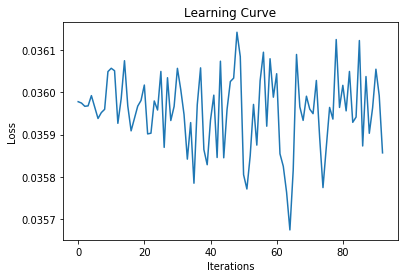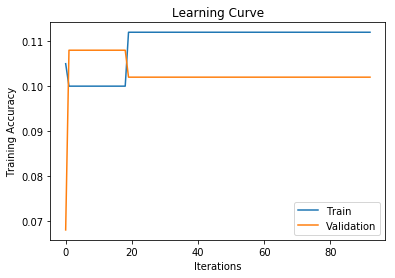Final Training Accuracy: 0.112
Final Validation Accuracy: 0.102